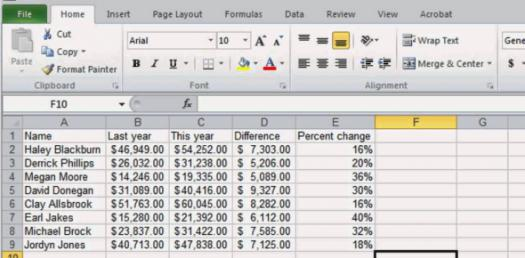# Spreadsheet Basic Functions! Trivia Quiz

10 Questions | Total Attempts: 351SettingsDo you have a perfect understanding of basic spreadsheet functions? Spreadsheets are mostly used to create budgets, produce graphs and charts, and for storing and sorting data in many organizations. This quiz is designed to assess mastery in the area of spreadsheet fundamentals. Do give it a try and get to see if you might need a refresher course when it comes to using this software application.

• 1.
What function should Eva use to calculate her final grade if her exam counts 25%, her test grades count 25% and her daily work count 50%?
• A.

Average

• B.

If

• C.

Max

• D.

Min

• 2.
What function will display the word “Yes” when the value in cell A1 is greater than the value in Cell B1 and “No” when otherwise?
• A.

If

• B.

Lookup

• C.

Max

• D.

Min

• 3.
Which function can an accounting department use in Cell A2 that will result in the current date?
• A.

Count

• B.

CountA

• C.

DAYS360

• D.

NOW

• 4.
What function will calculate the total cost of merchandise plus the tax calculated at a rate of 7.5% of the total merchandise cost?
• A.

Average

• B.

Max

• C.

Min

• D.

Sum

• 5.
Which function can a credit card company use to determine the time that has elapsed since a customer’s payment was received?
• A.

Count

• B.

CountA

• C.

DAYS360

• D.

NOW

• 6.
A spreadsheet is created with drop-down items that a user can select from. This is an example of which function?
• A.

Count

• B.

Fill Series

• C.

List

• D.

Lookup

• 7.
What function should a sales manager use to indicate whether a salesperson met the goal of \$6,000 sales this month?
• A.

Count

• B.

CountA

• C.

Fill Series

• D.

If

• 8.
What operation will Eve use to keep rows and columns visible so that they don’t scroll off the screen as she moves around the worksheet?
• A.

Embed

• B.

Fill Series

• C.

Freeze Panes

• D.

• 9.
What procedure should be followed to ensure that an object does NOT change in a word processing document when it is edited in the spreadsheet of its original creation?
• A.

Embed

• B.

Import

• C.

• D.

Target

• 10.
Mr. Evans sorted the student-athletes in order by GPA, last name, first name, and homeroom. GPA is an example of which part of the sort operation?
• A.

Ascending

• B.

Descending

• C.

Primary

• D.

Secondary

Related TopicsBack to top